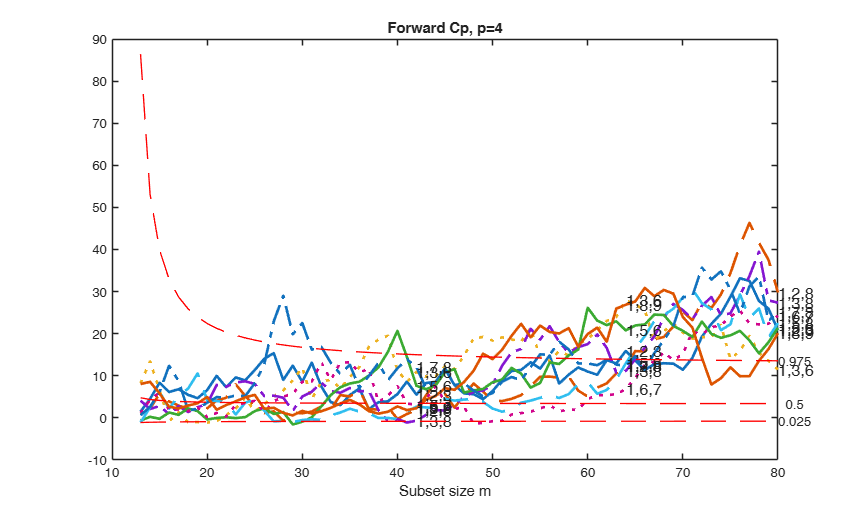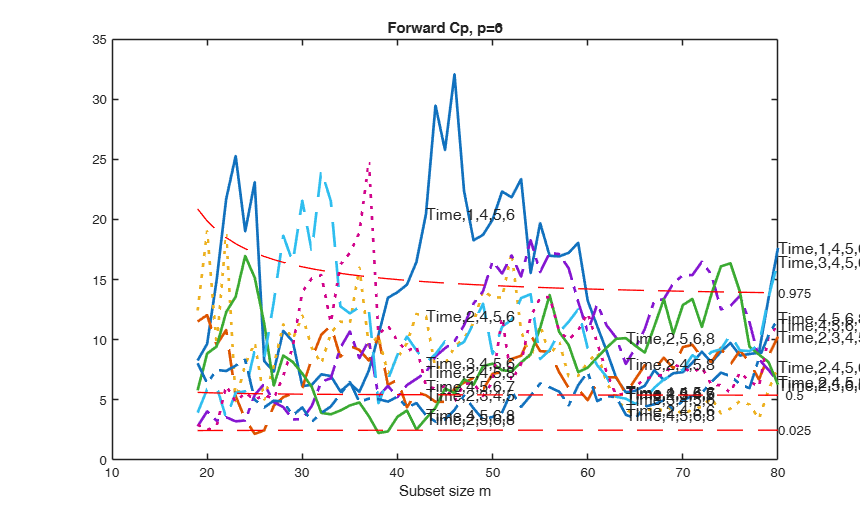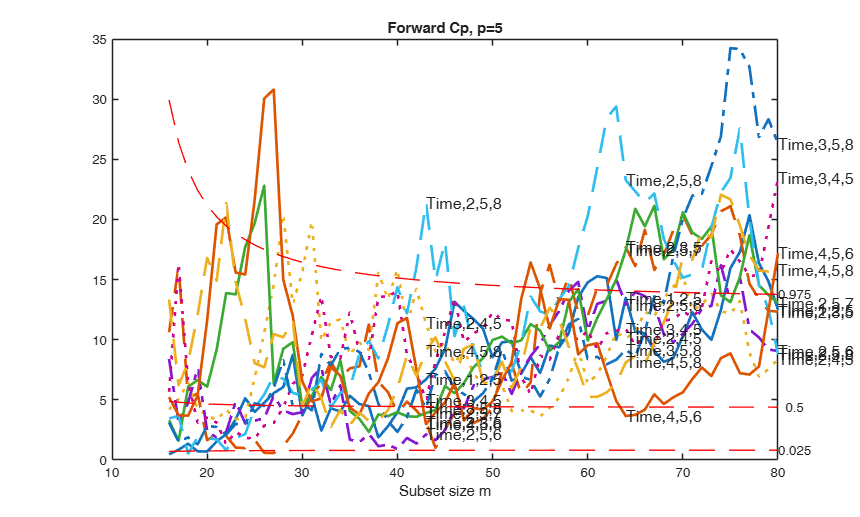# FSRcp

FSRcp monitors Cp and AIC for all models of interest of size smallp

## Syntax

• outCp=FSRcp(y,X,smallp)example
• outCp=FSRcp(y,X,smallp,Name,Value)example

## Description

 outCp =FSRcp(y, X, smallp) FSRcp with all default options.

 outCp =FSRcp(y, X, smallp, Name, Value) FSRcp with optional arguments.

## Examples

expand all

### FSRcp with all default options.

Extract the best models of size 4.

% Common part to all examples: load Ozone dataset.
% Transform the response using logs
X(:,end)=log(X(:,end));
X=[(-40:39)' X];
% Define y
y=X(:,end);
% Define X
X=X(:,1:end-1);
smallp=4;
[Cpmon]=FSRcp(y,X,smallp);

###FSRcp with optional arguments.

Extract the best models of size 4, and show the plot of forward Cp.

X=load('ozone.txt');
% Transform the response using logs
X(:,end)=log(X(:,end));
X=[(-40:39)' X];
% Define y
y=X(:,end);
% Define X
X=X(:,1:end-1);
smallp=4;
[outCp]=FSRcp(y,X,smallp,'plots',1);
Warning: Rank deficient, rank = 3, tol =  2.056162e-11.
Warning: Rank deficient, rank = 3, tol =  2.512148e-11.
Warning: Rank deficient, rank = 3, tol =  2.997602e-11.
Warning: Rank deficient, rank = 3, tol =  2.056162e-11.
Warning: Rank deficient, rank = 3, tol =  2.512148e-11.
Warning: Rank deficient, rank = 3, tol =  2.997602e-11.## Related Examples

expand all

### Use labels defined by the user.

Extract the best models of size 4 and show the plot of Cp. All the default options are used, apart from labels, therefore the plot of Cp and the output matrix Cpmon.MAL only contains the searches associated with the smallest 3 values of Cp in the last 16 steps of the search.

X=load('ozone.txt');
% Transform the response using logs
X(:,end)=log(X(:,end));
X=[(-40:39)' X];
% Define y
y=X(:,end);
% Define X
X=X(:,1:end-1);
smallp=4;
labels={'Time','1','2','3','4','5','6','7','8'};
[Cpmon]=FSRcp(y,X,smallp,'plots',1,'labels',labels);

### Extract and show the trajectories of all models of size 4 of Cp.

Notice that in this last case the forward plot becomes unreadable.

X=load('ozone.txt');
% Transform the response using logs
X(:,end)=log(X(:,end));
X=[(-40:39)' X];
% Define y
y=X(:,end);
% Define X
X=X(:,1:end-1);
smallp=4;
[Cpmon]=FSRcp(y,X,smallp,'plots',1,'fin_step','');

### Extract the best models of size 5 and plot monitoring of Cp.

Extract 1000 subsets to initialize the search and use labels defined by the user.

X=load('ozone.txt');
% Transform the response using logs
X(:,end)=log(X(:,end));
X=[(-40:39)' X];
% Define y
y=X(:,end);
% Define X
X=X(:,1:end-1);
smallp=5;
labels={'Time','1','2','3','4','5','6','7','8'};
[Cpmon]=FSRcp(y,X,smallp,'nsamp',1000,'plots',1,'labels',labels);

###Extract the best models of size 6 and 5 and plot monitoring of Cp.

Extract 1000 subsets to initialize the search andse labels defined by the user. Exclude the searches of the models which were unacceptable for smallp=5.

X=load('ozone.txt');
% Transform the response using logs
X(:,end)=log(X(:,end));
X=[(-40:39)' X];
% Define y
y=X(:,end);
% Define X
X=X(:,1:end-1);
smallp=6;
labels={'Time','1','2','3','4','5','6','7','8'};
[Cpmon6]=FSRcp(y,X,smallp,'nsamp',1000,'plots',1,'labels',labels);
smallp=5;
[Cpmon5]=FSRcp(y,X,smallp,'nsamp',1000,'Excl',Cpmon6.Ajout,'plots',1,'labels',labels);### Customizing the graphical options.

In the following example we play with the graphical options

X=load('ozone.txt');
% Transform the response using logs
X(:,end)=log(X(:,end));
X=[(-40:39)' X];
% Define y
y=X(:,end);
% Define X
X=X(:,1:end-1);
labels={'Time','1','2','3','4','5','6','7','8'};
smallp=5;
[Cpmon]=FSRcp(y,X,smallp,'plots',1,'labels',labels,'xlimx',[40 80],'lwdenv',5,'lwd',4,'FontSize',25,'SizeAxesNum',20);

## Input Arguments

### y — Response variable. Vector.

A vector with n elements that contains the response variable.

Missing values (NaN's) and infinite values (Inf's) are allowed, since observations (rows) with missing or infinite values will automatically be excluded from the computations.

Data Types: single| double

### X — Predictor variables. Matrix.

Data matrix of explanatory variables (also called 'regressors') of dimension (n x (bigP-1)).

The intercept will be added in automatic way, so that the dimension of the full model is bigP Rows of X represent observations, and columns represent variables. Missing values (NaN's) and infinite values (Inf's) are allowed, since observations (rows) with missing or infinite values will automatically be excluded from the computations.

Data Types: single| double

### smallp — number of variables in the reduced models. Scalar.

Scalar which specifies the number of variables in the reduced models which will be considered. For example if smallp=3, all possible subsets containing 2 columns of matrix X will be considered. Notice that the dimension of each submodel is 3 because to each submodel the column of ones is added automatically.

Data Types: single| double

### Name-Value Pair Arguments

Specify optional comma-separated pairs of Name,Value arguments. Name is the argument name and Value is the corresponding value. Name must appear inside single quotes (' '). You can specify several name and value pair arguments in any order as  Name1,Value1,...,NameN,ValueN.

Example:  'intercept',false , 'nocheck',true , 'h',3 , 'lms',1 , 'lms',1 , 'nsamp',1000 , 'init',100 starts monitoring from step m=100 , 'aic',1 , 'labels',{'Time','1','2','3','4','5','6','7','8'} , 'fin_step',1 , 'first_k',5 , 'Excl',[23; 24] , 'ExclThresh',0.6 , 'plots',1 , 'labout',1 , 'quant',0.1 , 'steps',[4 8] , 'titl','my title' , 'labx','my label' , 'laby','my label' , 'xlimx',[0 1] , 'ylimx',[0 1] , 'linewidth',6 , 'lwdenv',6 , 'FontSize',20 , 'SizeAxesNum',30 , 'selunitcolor',{'b';'g';'r'} , 'selunittype',{'-';'--';':';'-.'} 

### intercept —Indicator for constant term.true (default) | false.

Indicator for the constant term (intercept) in the fit, specified as the comma-separated pair consisting of 'Intercept' and either true to include or false to remove the constant term from the model.

Example:  'intercept',false 

Data Types: boolean

### nocheck —Check input arguments.boolean.

If nocheck is equal to true no check is performed on matrix y and matrix X. Note that y and X are left unchanged. In other words the additioanl column of ones for the intercept is not added. As default nocheck=true.

Example:  'nocheck',true 

Data Types: boolean

### h —number of observations that have determined the least trimmed squares estimator.integer.

h is an integer greater than smallp+1 but smaller then n. The default value of h is [(n+smallp+1)/2]

Example:  'h',3 

Data Types: double

### lms —Criterion to use to find the initlal subset to initialize the search.scalar.

If lms=1 (default) Least Median of Squares is computed, else Least Trimmed of Squares is computed.

Example:  'lms',1 

Data Types: double

### nomes —Displaying time message.scalar.

If nomes is equal to 1 (default) no message about estimated time to compute LMS (LTS) for each considered model is displayed, else a message about estimated time is displayed.

Example:  'lms',1 

Data Types: double

### nsamp —Number of subsamples which will be extracted to find the robust estimator.scalar.

If nsamp=0 all subsets will be extracted.

They will be (n choose smallp).

Remark: if the number of all possible subset is <1000 the default is to extract all subsets otherwise just 1000.

Example:  'nsamp',1000 

Data Types: double

### init —Search initialization.scalar.

It specifies the initial subset size to start monitoring the required quantities, if init is not specified it will be set equal to smallp+1, if the sample size is smaller than 40;

min(3*smallp+1,floor(0.5*(n+smallp+1))), otherwise.

Example:  'init',100 starts monitoring from step m=100 

Data Types: double

### aic —Akaike's information criterion.scalar.

If aic=1 the value of AIC is also stored in each step of the search else (default) only Mallows Cp is stored

Example:  'aic',1 

Data Types: double

### labels —names of the explanatory variables.cell array of strings.

Cell array of strings of length bigP-1 containing the names of the explanatory variables. If labels is a missing value the following sequence of strings will be automatically created for X (1,2,3,4,5,6,7,8,9,A,B,C,D,E,E,G,H,I,J,K,...,Z)

Example:  'labels',{'Time','1','2','3','4','5','6','7','8'} 

Data Types: cell

### fin_step —portion of the search which has to be monitored to choose the best models.scalar.

If fin_step is an integer greater or equal 1, it refers to the number of steps.

For example if fin_step=10 the program considers the last 10 steps to choose the best models.

If fin_step is a real number alpha (0<alpha<0.5) in the interval (0 0.5] than the program considers the last round(n*alpha) steps. As default fin_step=round(n*0.2) that is the last 20% of the steps are considered.

Remark1: the number of best models to consider is controlled by scalar first_k (see below).

Remark2: if fin_step is an empty value, no selection is done and all trajectories of Cp are displayed (in this case the value of first_k below is ignored, all models are considered of interest and output matrix outCp.Ajout is equal to an empty value).

Example:  'fin_step',1 

Data Types: double

### first_k —number of best models to consider in each of the last fin_step.scalar.

For example if first_k=5 in each of the last fin_step, the models which had the 5 smallest values of Cp are considered.

As default first_k=3

Example:  'first_k',5 

Data Types: double

### Excl —Matrix which contains the models which surely do not have to be considered.matrix.

As default Excl='' For example if smallp=3, bigP=6 and Excl = [23; 24; 27]; the three models 23, 24, and 27 are skipped

Example:  'Excl',[23; 24] 

Data Types: double

### ExclThresh —Exclusion threshold.scalar.

Exclusion threshold associated to the upper percentage point of the F distribution of Cp which defines the threshold for declaring models as unuseful.

The default value of ExclThresh is 0.99999 that is the models whose minimum value of Cp in the part of the search defined by fin_step is above ExclThresh are stored in output matrix outCp.Ajout. Notice that ExclThresh must be smaller than 1

Example:  'ExclThresh',0.6 

Data Types: double

### plots —Plot on the screen.scalar.

If plots==1 a plot is created on the screen which contains the trajectories of Cp monitored along the search with confidence bands If plots==2 two plots are generated. The first contains the trajectories of Cp monitored along the search with confidence bands. The second contains the trajectories of AIC monitored along the search else (default) no plot is shown on the screen

Example:  'plots',1 

Data Types: double

### labout —If labout=1 the output LABOUT contains the list of models whose Cp values are inacceptable.scalar.

Default: no model is created.

Remark: the options below only work if plots is equal 1.

Example:  'labout',1 

Data Types: double

### quant —It specifies the quantiles which are used to produce Cp envelopes.vector.

The elements of quant are numbers between 0 and 1. The default value of quant is quant=[0.025 0.5 0.975];

Example:  'quant',0.1 

Data Types: double

### steps —Steps to add labels.vector.

It specifies in which steps of the plot which monitors Cp it is necessary to include the labels of the models which have been previously chosen.

The default is to write the labels of the models in steps round([n*0.6 n*0.8 n]);

Example:  'steps',[4 8] 

Data Types: double

### titl —a label for the title.character.

default is ['Forward Cp' p= num2str(smallp)]

Example:  'titl','my title' 

Data Types: char

### labx —a label for the x-axis.character.

default: 'Subset size m'

Example:  'labx','my label' 

Data Types: double

### laby —a label for the y-axis.character.

default:''

Example:  'laby','my label' 

Data Types: char

### xlimx —minimum and maximum on the x axis.vector.

Default value is '' (automatic scale)

Example:  'xlimx',[0 1] 

Data Types: double

### ylimy —minimum and maximum on the y axis.vector.

Default value is '' (automatic scale)

Example:  'ylimx',[0 1] 

Data Types: double

### lwd —linewidth of the curves which contain the score test.scalar.

Default line width=2

Example:  'linewidth',6 

Data Types: double

### lwdenv —width of the lines associated with the envelopes.scalar.

Default is lwdenv=1

Example:  'lwdenv',6 

Data Types: double

### FontSize —font size of the labels of the axes and of the labels inside the plot.scalar.

Default value is 12

Example:  'FontSize',20 

Data Types: double

### SizeAxesNum —size of the numbers of the axes.scalar.

Default value is 10

Example:  'SizeAxesNum',30 

Data Types: double

### selunitcolor —colors to be used for the Cp trajectories.cell array of strings.

If selunittype is not specified or if it is an empty value default Matlab colors are used.

Example:  'selunitcolor',{'b';'g';'r'} 

Data Types: cell

### selunittype —line types of the Cp trajectories.cell array of strings.

If selunittype is not specified or if it is an empty value all possible line styles are used.

Example:  'selunittype',{'-';'--';':';'-.'} 

Data Types: cell

## Output Arguments

### outCp — description Structure

containing the following fields:

Value Description
MAL

(n-init+1) x (k+1) matrix.

Mallows Cp monitored along the search:

1st col is fwd search index;

2nd col is associated with first selected model;

3rd col is associated with second selected model;

...;

(k+1)th col is associated with k-th selected model.

Notice that k<=(n choose smallp) and that all models contain the constant.

AIC

(n-init+1) x (k+1) matrix.

AIC monitored along the search:

1st col is fwd search index;

2nd col is associated with first selected model;

3rd col is associated with second selected model;

...;

(k+1)th col is associated with k-th selected model.

Remark 1: k<=(n choose smallp).

Remark 2: all models contain the constant.

Remark 3: matrix AIC is produced only if input option aic=1.

UnAll

cell of dimension k. Each element of the cell is a (n-init) x 11 matrix containing the unit(s) included in the subset at each step of the search.

REMARK: in every step the new subset is compared with the old subset. Un contains the unit(s) present in the new subset but not in the old one.

LAB

cell array of strings of length k containing the labels of the models which have been extracted. First element of LAB is associated with second column of matrix MAL...

Ajout

numeric matrix which contains the list of the models whose Cp values are inacceptable.

The number of columns of matrix Ajout is equal to smallp-1 This information is useful because in this way it is possible to skip the computation of the submodels of the rows of matrix Ajout.

For example if smallp=3, bigP=6 and Ajout = [ 23; 24; 27 ] the three models 23, 24, and 27 always have Cp values much greater than the threshold (that is variables 2,3,4,7 are considered unimportant).

LABout

cell array of strings which contains as strings the list of models which are unacceptable.